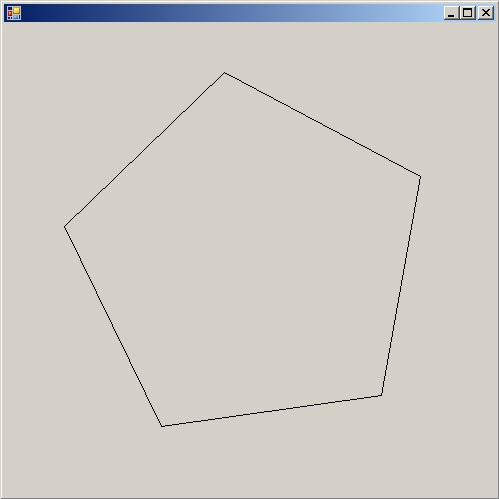Learn to Code
John F. Dumas

Why Learn C#?

► Unlike languages such as C or C++, C# comes with everything you need to build gui programs right out-of-the-box.

Here's an example program that shows a rotating, 5-sided polygon. Observe that only about 60-70 lines of code are required to create this application. Here's the source and a screenshot of the running application (file: test.cs):

• ```using System.Windows.Forms;
using System.Drawing;
using System;

public class Test : Form
{
public Test()
{
Size   = new Size(500, 500);
Paint += new PaintEventHandler(DoRender);

mTimer.Tick += new System.EventHandler(
delegate { if(++mDegrees == 360) mDegrees = 0; Refresh(); }
);

mTimer.Interval = 25;
mTimer.Enabled  = true;
}

private void DoRender(object sender, PaintEventArgs e)
{
e.Graphics.Clear(BackColor);

int xCenter = ClientSize.Width  / 2;
int yCenter = ClientSize.Height / 2;

int radius = (int)(.8 * Math.Min(xCenter, yCenter) + 0.5);

int xFirst = 0, yFirst = 0, xOld = 0, yOld = 0;

for(int i = 0, degrees = mDegrees; i < N_SIDES; i ++, degrees += STEP)
{
double angle = degrees * (Math.PI / 180.0);

int x = (int)Math.Round(xCenter + radius * Math.Cos(angle));
int y = (int)Math.Round(yCenter + radius * Math.Sin(angle));

if(i == 0)
{
xFirst = x;
yFirst = y;
}
else
{
e.Graphics.DrawLine(Pens.Black, x, y, xOld, yOld);

if(i + 1 == N_SIDES)
e.Graphics.DrawLine(Pens.Black, x, y, xFirst, yFirst);
}

xOld = x;
yOld = y;
}
}

private const int N_SIDES = 5;
private const int STEP    = (360 / N_SIDES);

private int   mDegrees = 0;
private Timer mTimer   = new System.Windows.Forms.Timer();

static void Main()
{
Application.Run(new Test());
}
}
```

Results:► Being part of the .Net framework means that C# has access to a wealth of tools and libraries.

► C# is popular. As of July, 2021, the TIOBE Index of programming popularity was:

1. C
2. Java
3. Python
4. C++
5. C#

► Object oriented, very similar to Java but with some substantial improvements such as Properties and LINQ.

► The Unity game engine has a .Net api so you can write your games in C#. Also, Unity's editor application's under interface is written in C#.

► ONLINE RESOURCES

► BOOKS

Back to Examples Index

© John F. Dumas | johnfdumas@gmail.com | main page | top of page